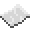# math.modf

Return the integer and fractional parts of number.ExamplePrint the integral and fractional parts of user input
Read a single number from the user, then print its integral and fractional parts.
Code
```<nowiki>
</nowiki>
```
Output Depends. If the user wrote `5.2`, the numbers printed will be `5 0.2`

math.modf
Function
Syntax
math.modf(
• number : number
)

Returns number integral, number fractional
API math
Source Lua (source)